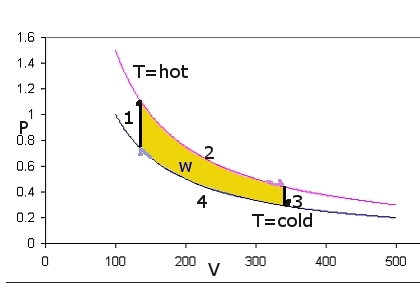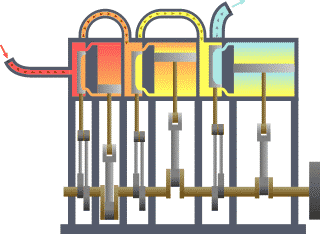Skip to main content

# 20.7: Thermodynamics Provides Insight into the Conversion of Heat into Work

Heat and work are both forms of transferring energy and under the right circumstance one form may be transformed into the other. However, the second law of thermodynamics puts a limitation on this. To go from work to heat is called dissipation and there is no limitation on this at all. In fact it was through dissipation (by friction) that we discovered that heat and work were both forms of transferring energy.

## The Carnot Cycle

To go the other way is much harder. We can see that if we consider a circular reversible path in an ideal PV diagram in Figure $$\PageIndex{1}$$; we start bottom left. The path consists of four steps:

1. Process 1 is an isochoric heating from $$T_c$$ to $$T_h$$ (with constant $$C_v$$): $q_1= +\dfrac{C_v}{ΔT} \label{q1}$
2. Process 2 is an isothermal expansion at $$T_h$$: $q_2=q_{hot} =-w$ and we get work out (i.e., negative work): $w_{hot} =-RT_h \ln \left( \dfrac{V_2}{V_1} \right)$
3. Process 3 is an isochoric cooling from $$T_h$$ to $$T_c$$ (with constant $$C_v$$): $q_3= -\dfrac{C_v}{ΔT} \label{q3}$
4. Process 4 is an isothermal compression at $$T_c$$: $q_4=q_{cold} =-w$ and we must put work in (i.e., positive work): $w_{cold} =+RT_c \ln \left( \dfrac{V_2}{V_1} \right)$Figure $$\PageIndex{1}$$: Carnot Cycle

The total four-step process produces work because $$w_{hot} > w_{cold}$$. The work is the integral under the upper isotherm minus the one under the lower curve, i.e.the surface area in between. Notice that this yellow area vanishes if $$T_{hot}= T_{cold}$$. Obviously how cold the cold side is of great importance!. The amount of work is also equal to the difference in the heat picked up at high temperature qhot and dumped at low temperature $$q_{cold}$$. The isochoric heats cancel. The problem is that $$q_{cold}$$ is only zero if the cold temperature is 0 K. That means that we can never get all the heat we pick up at high temperatures to come out as work.

Sadi Carnot

Sadi Carnot was a French engineer at the beginning of the 19th century. He considered a cyclic process involving a cylinder filled with gas. This cycle the Carnot cycle contributed greatly to the development of thermodymanics and the improvement of the steam engine. Carnot demonstrated that the cold temperature on the right is as important as the heat source on the left in defining the possible efficiency of a heat engineFigure $$\PageIndex{2}$$: Animation of a typical vertical triple-expansion engine. (CC SA-BY 2.5; Emoscopes).

## Efficiency

We can use the state function S to calculate how efficiently we can use heat to get useful work done. As the path is circular the circular integrals for both $$U$$ and $$S$$ are zero since they are state variables. This means that

$0 = \Delta S = \int_{1+2+3+4} \dfrac{\delta q}{T} = \left[ \dfrac{q_{rev}}{T}\right]_{hot} + \left[ \dfrac{q_{rev}}{T}\right]_{cold}$

since the heats from the two isochore steps cancel via Equations $$\ref{q1}$$ and $$\ref{q3}$$ (i.e., $$q_1 = -q_3$$). Therefore

$\dfrac{q_{cold}}{q_{hot}} = \dfrac{T_{cold}}{T_{hot}}$

Of course we spend good money on the fuel to start the cycle by heating things up. So how much work do we get for the heat we put in? If we define the efficiency of our heat engine as

$\eta ≡-\dfrac{w}{q_{hot}}$

we get that

$η= 1+ \dfrac{q_{cold}}{q_{hot}} = 1-\dfrac{T_c}{T_h} \label{eff}$

As you see we can only get full efficiency if $$T_{cold}$$ is 0 K, which is never (i.e., we always waste energy).

Another implication is that if $$T_c = T_h$$ then no work can be obtained. no matter how much energy is available in the from of heat. Or in other words, if one dissipates work into heat isothermally, none of it can be retrieved.

Equation $$\ref{eff}$$ is not very forgiving at all. Just imaging that you have a heat source of say 400 K (a superheated pool of water, e.g. a geyser) and you are dumping in the river at room temperature 300 K. The best efficiency you'll ever get is:

$η= = 1-\dfrac{300}{400}= 24\%$

Sadly, you'd be dumping three quarters of your energy as heat in the river.. (And that is best case scenario. There are always more losses, e.g. due to friction). The arrow saying $$Q_C$$ in Figure $$\PageIndex{3}$$ should then be three times as fat as the one that says W.Figure $$\PageIndex{3}$$: Carnot engine diagram (modern) - where an amount of heat QH flows from a high temperature THfurnace through the fluid of the "working body" (working substance) and the remaining heat QC flows into the cold sink TC, thus forcing the working substance to do mechanical work W on the surroundings, via cycles of contractions and expansions. (Public Domain; Eric Gaba).

## Heat pumps

So getting our work from heat is hard and always less than 100% successful. The other way around should be easy. After all, we can dissipate work into heat freely even under isothermal conditions!

What happens if we let the heat engine run backwards? Consider reversing all the flows in the above diagram. Obviously we must put in work to make the cycle run in reverse. The heat will now flow from cold to hot, say from your cold garden into your nice and warm apartment. The amount of heat you get in your humble abode will be the sum of all the work (say 100 Joules) you dissipate plus the heat you pumped out of the garden (say 300 Joules). Thus if you are willing to pay for the energy you dissipate (100 Joules), you may well end up with a total of 400 Joules of heat in your apartment!.

Obviously if it is heat you after this is a better deal than just dissipating the work in your apartment (by burning some oil). Then you'd only get 100J for your precious buck.

Dissipating it as electrical heating is even worse because you would

1. first burn (a lot more!) oil to generate heat
2. use this heat to produce electrical work at great expense because a lot of the heats gets dumped at the low temperature side (the river or so)
3. dissipate the work again in your apartment (without using it to pump any heat out of the garden)

I heat my apartment electrically.

To my defense I should add that I do not have a garden to pump heat from and I certainly do not have the (expensive) system of pipes, pumps and exchangers that would be required to make a heat pump work.

I do have a refrigerator. This is a heat pump too. It heats my room by pumping heat from its innards into my room. If I keep the door open, however, all it does is dissipate precious electrical work, because the pumped heat will flow back into its innards and spoil my milk.

• Was this article helpful?